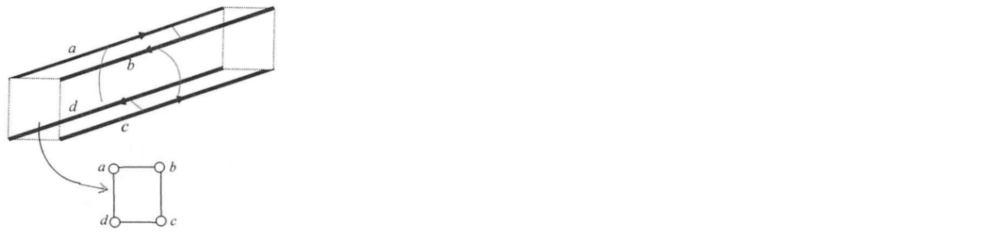# Problem: Four parallel wires, a, b, c, d, are placed at the corners of a square glass beams as shown. (The insert shows the end view from the left). Wires a and c carry parallel currents to the right and wires b and d to the left. Study all possible interactions between the currents (six interactions altogether) and tell: How many attractive interactions you find? _____ How many repulsive ones? _____

###### Problem Details

Four parallel wires, a, b, c, d, are placed at the corners of a square glass beams as shown. (The insert shows the end view from the left). Wires a and c carry parallel currents to the right and wires b and d to the left. Study all possible interactions between the currents (six interactions altogether) and tell:

How many attractive interactions you find? _____

How many repulsive ones? _____